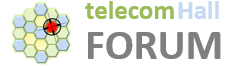# 5G frames structure

I am still confused on frames structure of 5g

radio frames ,scs, slots, symbols, resource elements, PRB, RB, REG, CRB, CP

Below Image should give you an over view of SCS,SLOTS for NR

Thanks for sharing… I understand it…

Brother,
Same thing like 4G. (1 Frame is of 10ms = 10 SubFrames( SFs ))
1 SF = 1ms (Differs as per the Numerology/SubcarrierSpacing (SCS)
Next change comes here where 1SF or slot (for scs 15Khz( 0 numerology )) = 14 symbols(Time Domain) and 12 Sub-Carries(Freq domain) Called as (ResourceBlock or Common Resource Blocks( CRB )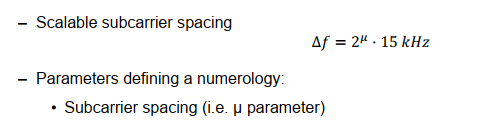Based on the Numerology 0(15Khz),1(30Khz),2(60Khz),3(120Khz) or 4(240Khz) No of Slots changes per SubFrame(SF).
One thing to remember always is that Irrespective of Numerology, We have 14 symbols per Slot and only changes is TS duration and No of slots per SF according to numerology.
From the below snap, you might get clear with FS.

REG means Resource Element Group same as LTE which is used to defined control region only.
Major difference comes in REs and REGs.
In LTE, 1 CCE=9 REGs in which 1 REG = 4 REs, So total we had 36 REs in 1 CCE(9x4=36 REs) .
whereas in
5G, 1 CCE=6 REGs in which 1 REG= 12 REs in freq Domain(which is also called as RB, Little confusing right, Remember 12 SCs in freq and 1 symbol in Time domain we use to define CCE in 5G) So total we have 72 REs in 1 CCE(6x12=72 REs)

CP we have same for all Numerology. Only we use Extended CP for 2(60Khz) Numerology in which we have 12 symbols instead of 14. You can check above table for the same.

1 Like

Thanks for sharing now I understanding well in 5G frames structure… I how CCE for aggregation level works in 5g…

Dear,
Below is the CCE detailed information.

PDCCH CCE Aggregation level:-
The number of Resource Elements (RE’s) of a CORESET that are required to carry a PDCCH DCI message is called “Aggregation level”, It is expressed in terms on CCE’s (control channel elements).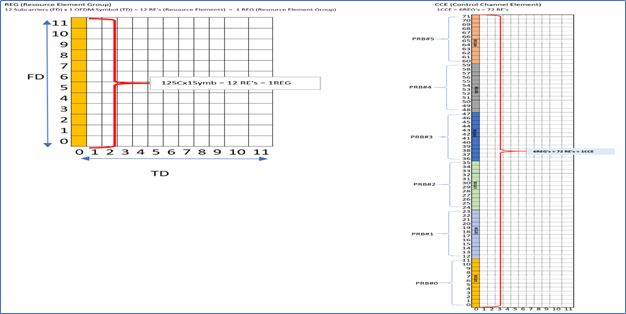Number of REG’s/CCE’s that compose a PDCCH candidate is determined by the CCE Aggregation level, Aggregation level and number of CCE’s per aggregation are matched one to one.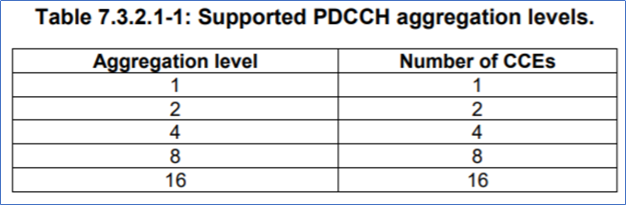Control Resource Set(CORESET) Resource Configuration: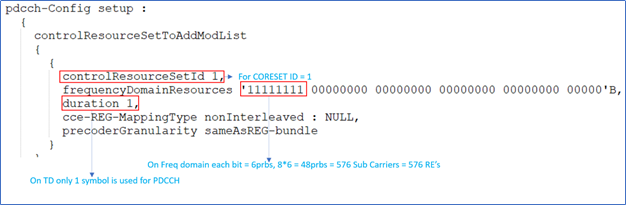Based on the above configuration below is an example applying CCE Aggregation level for a DCI format.

1REG = 12SC’s X 1symbol = 12 RE’s

1CCE = 6 REG’s = 72 RE’s (54 REs are used for PDCCH, 18 RE’s are used for DMRS)

Total PRBs in the CORESET = 48

Total number of CORESET Resource elements = 576

Number of DMRS REs = 18 * 8 = 144

PDCCH RE’s = 576 - 144 = 432 (meaning In this case PDCCH candidate up to maximum Aggregation level 8 can be configured, Aggregation Level 16 is not possible in this case).

Following are 5 different PDCCH CCE aggregation levels supported in 5G NR, Aggregation Level 1, 2, 4 , 8 & 16 Each of these aggregation numbers specify the number of CCE’s (RE’s) that are required to carry a PDCCH DCI message(TS 38.213)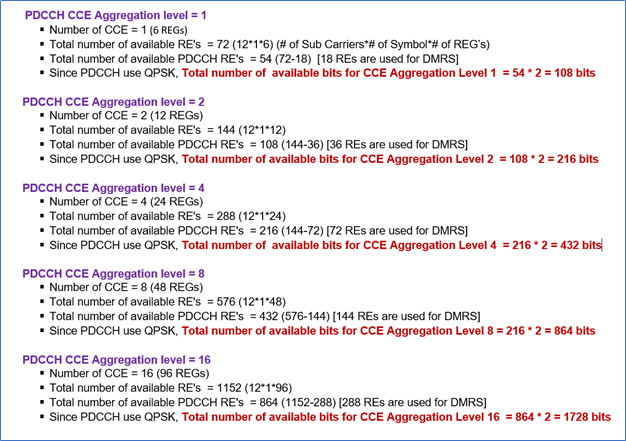• Number of bits (Payload) of the DCI formats are less than the number of bits per each CCE aggregation level (as shown above).
• For example, Size of DCI format 1_1 scrambled with a C-RNTI for FR2 100Mhz (66RBs), the total number of bits after packing all the fields is 79 bits (referenced 38.212, 7.3.1.2.2) and after adding the 24 bit CRC, total payload size of the DCI 1_1 is 103 bits,

shown in below snap shot.

• In above example since DCI Format 1_1 is scrambled with a C-RNTI, assuming Aggregation level 2 is applied, meaning 103 bits payload of the DCI Format 1_1 is rate matched and bloated to 216 bits (applied aggregation level 2 = 2 CCE’s) giving a very good forward error correction protection for efficient decoding of PDCCH and derive the DCI message bits successfully.
• As per specification it mandates all DCI’s scrambled with a Common RNTI such as SI, RAR, Paging etc that have PDCCH candidates allocated in Common search space should always use highest Aggregation level 4 or 8.
• DCI’s scrambled with C-RNTI that have PDCCH candidates in UE Specific search space could use lower Aggregation level, This information is communicated to the UE via RRC Reconfigure message.

This is how “CCE” aggregation level works in 5G(NR). hope this will helpful you to understand this topic.